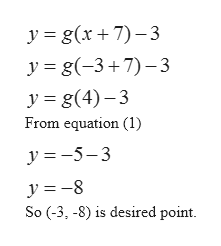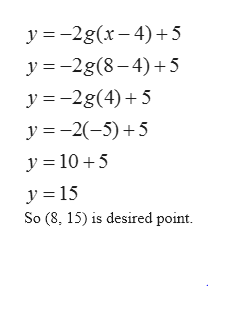# Suppose (4,-5) is a point on the graph of y=g(x)what is the point on the graph of y=g(x+7)-3y=-2g(x-4)+5and y=g(2x+10)

Question
18 views

Suppose (4,-5) is a point on the graph of y=g(x)

what is the point on the graph of y=g(x+7)-3

y=-2g(x-4)+5

and y=g(2x+10)

check_circle

Step 1

Given (4, -5) lies on graph y=g(x) so

Step 2

Now for finding the point lies on y=g(x+7)-3, we choose x in such a way that we get x+7=4 (i.e. g(4)). So choose x=4-7= -3,help_outlineImage Transcriptionclosey g(x7)-3 y g(-3+7)-3 y g(4)-3 From equation (1) y =5-3 So (-3, -8) is desired point fullscreen
Step 3

Similarly, for y=-2g(x-4)+5, cho...help_outlineImage Transcriptionclosey 2g(x4)5 y 2g(8-4)5 y 2g(4)5 y 2(5)5 y 10 5 y 15 So (8, 15) is desired point fullscreen

### Want to see the full answer?

See Solution

#### Want to see this answer and more?

Solutions are written by subject experts who are available 24/7. Questions are typically answered within 1 hour.*

See Solution
*Response times may vary by subject and question.
Tagged in

### Other